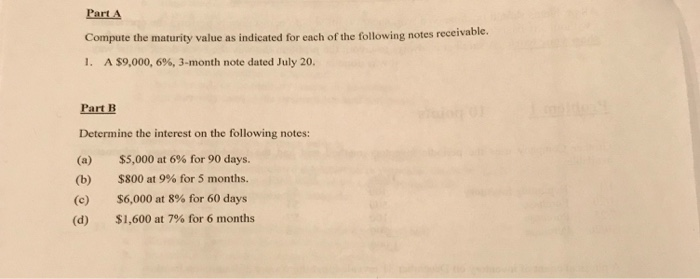### Create an Account

Home / Questions / Part A Compute the maturity value as indicated for each of the following notes receivable. 1. A \$9,0

# Part A Compute the maturity value as indicated for each of the following notes receivable. 1. A \$9,0Part A Compute the maturity value as indicated for each of the following notes receivable. 1. A \$9,000, 6%, 3-month note dated July 20. Part B Determine the interest on the following notes: \$5,000 at 6% for 90 days. (a) \$800 at 9% for 5 months. (b) \$6,000 at 8% for 60 days (c) \$1,600 at 7% for 6 months (d)

Apr 01 2020 View more View LessSubscribe To Get Solution